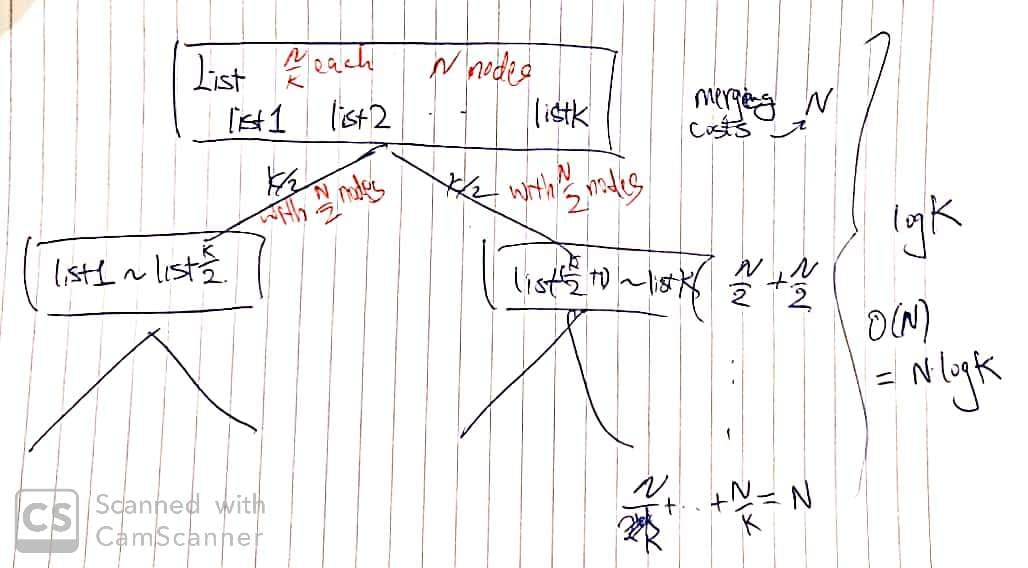# 23. Merge k Sorted Lists

Reference: LeetCode EPI 10.1
Difficulty: Hard

## Problem

Merge $k$ sorted linked lists and return it as one sorted list. Analyze and describe its complexity.

Example:

## Analysis

Focus on the third and fifth solution.

Test Case:

### Brute-Force

It is okay if $N$ is not too large.

• Traverse all the linked lists and collect the values of the nodes into an array. - $O(N)$
• Sort the array. - $O(N\log{N})$
• Traverse the array and make the linked list. - $O(N)$

Time: $O(N\log{N})$ where $N$ is the total number of nodes.
Space: $O(N)$ since we need an array and a new linked list.

### Compare One-By-One

(if $k$ is much less than $N$, $k$ is the number of lists)

Compare every $k$ nodes (head of every list) and get the smallest node.

Note:

• Use minIdx to record the location and to check if the list is empty.

Time: $O(kN)$ where $k$ is the number of linked lists. 311 ms
Space: $O(N)$ creating a new linked list. Or $O(1)$ if we apply an in-place method. Connect selected nodes instead of creating new nodes.

### Compare One-By-One (minPQ)

Use a minimum priority queue to store $k$ nodes. Pop the smallest node and offer its next node if it is not null.

Time: $O(N\log{k})$ 34 ms

• Initializing the priority queue takes $O(k\log{k})$
• Pop $N$ nodes from the priority queue takes $O(N\log{k})$

Space: $O(k)$ since priority queue stores $k$ nodes. $O(1)$ or $O(N)$ depends on the input $N$ and $k$ and whether we create a new linked list.

### Merge Lists One-By-One

We need to merge $k$ lists by merging $(k-1)$ times.

Note:

• mergeList(dummy.next, n) is thoughtful. At the beginning, dummy.next is null, but it does not matter.
• Alternatively, we can use the first place of the array to store merged list.

Time: $O(kN)$ 250 ms

• Merge two sorted lists in $O(n)$ time where $n$ is the total number of nodes in two lists. (worst case)
• To sum up we have: $O(\sum_{i=1}^{k-1}(i * \frac{N}{k} + \frac{N}{k}) = O(kN)$. (key: $n = \frac{N}{k}$)

Space: $O(1)$ since we merge in place.

### Merge Lists with Divide And Conquer

In effect, we don’t need to traverse most nodes many times repeatedly. We can divide lists in half until there is only one list. Merge them one by one to get the final result. It’s similar to mergesort.

Note:

• Recall of the left-leaning and right-leaning cases.
• The base case is thoughtful. lo > hi actually won’t occur. And lists[lo] won’t change other elements on the other side.
• lists.length == 0 condition is very important.
• Input case: [].

Time: $O(N\log{k})$ 2 ms
Space: $O(\log{k})$ if we use recursion (depth of the recursion tree).CommentJunhao Wang
a software engineering cat